# C.2 Bose-EINSTEIN Statistics

For Bosons the occupation number is not restricted, so one must sum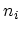over all integers in (C.12)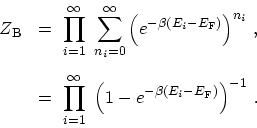(C.16)

The logarithm of (C.16) yields the thermodynamic potential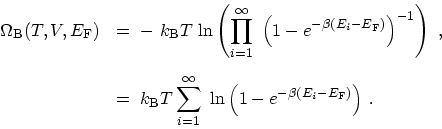(C.17)

The mean number of particles is obtained from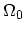by differentiating with respect to the FERMI energy, as in (C.5), by keeping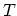and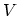(equivalently the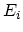) fixed. As a result, the mean number of Bosons is given by the Bose-EINSTEIN distribution function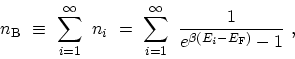(C.18)

whereis the mean occupation number in the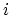th state. M. Pourfath: Numerical Study of Quantum Transport in Carbon Nanotube-Based Transistors